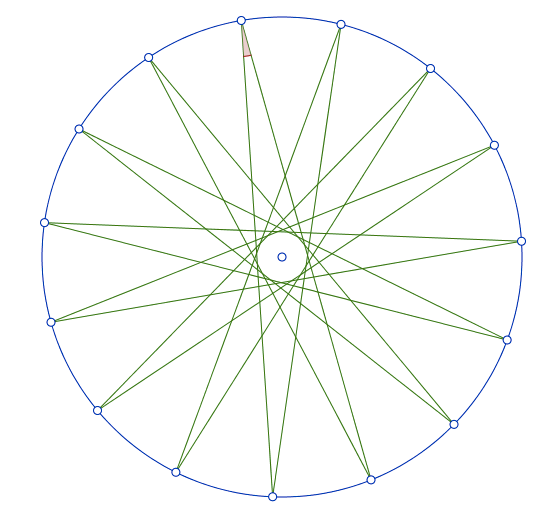Star Study - Integral Angles

Geometry Level 3Take $n$ points that are equally spaced on the circumference of a circle. For each point, connect it to the 2 points that are furtherest away from it, but not diametrically opposite to it. This forms an $n$ pointed star.

For how many integers $n \geq 5$ is the measure (in degrees) of an individual internal angle in an $n$ pointed star also an integer?

×